# Thread: Potential Function

1. ## Potential Function

I need help finishing this question. The original question and my work so far are attached.

Thanks

2. I'm with you thus far. Now deduce what phi(z) is inside psi(y,z), just as you found psi(y,z) inside f(x,y,z).

$\displaystyle \frac{\partial \psi}{\partial z} = \frac{\partial f}{\partial z} - 2xyz = 4z - 2ye^{2z}$

And now,

$\displaystyle \frac{\partial \phi}{\partial z} = \frac{\partial \psi}{\partial z} - (-2ye^{2z}) = 4z$

So

$\displaystyle \phi = 2z^2 + C$

Have to dash, but will add a pic later.

... Hi there. Yes, just in case a picture helps. Your work so far in balloon sculpture...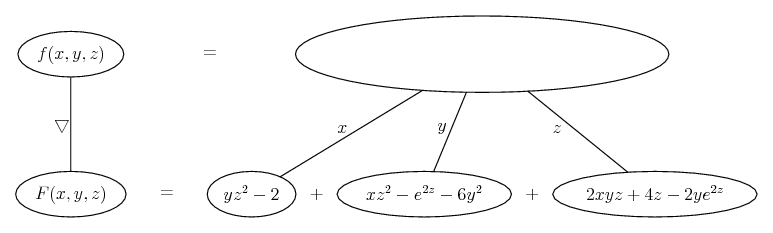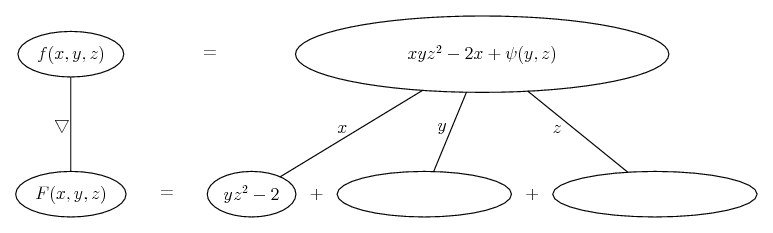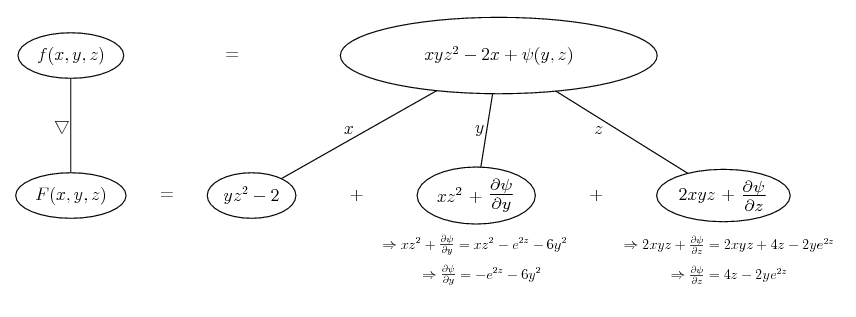... and why not determine psi similarly...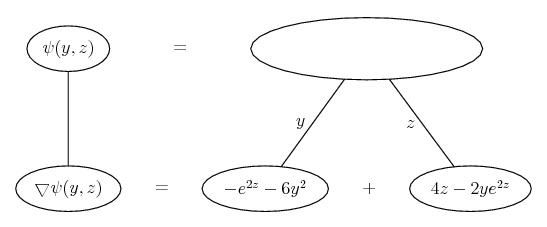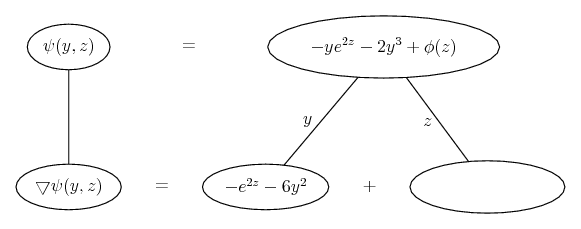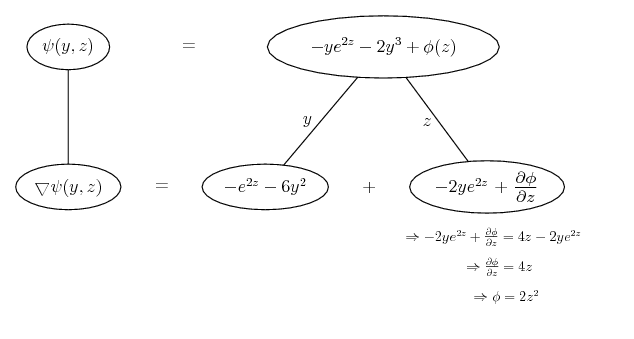__________________________________________

Don't integrate - balloontegrate!

Balloon Calculus; standard integrals, derivatives and methods

Balloon Calculus Drawing with LaTeX and Asymptote!

3. Thanks for the help. The picture really helps.

#### Search Tags

function, potential